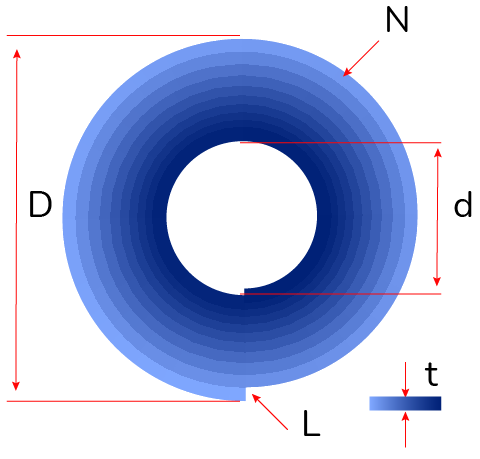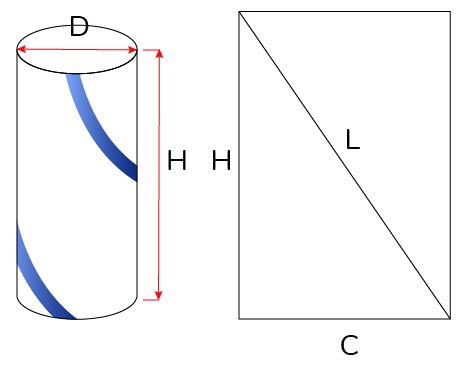# Spiral Length Calculator

Created by Joanna Śmietańska, PhD candidate
Reviewed by Dominik Czernia, PhD and Steven Wooding
Last updated: Jun 05, 2023

In everyday life, you can use this spiral length calculator to compute the length of all objects that appear in a rolled-up form: rolls of paper, tape, films, and so on.

The definition of spiral tells us that it is a trajectory of a point moving around a center point while moving continuously away from or towards it. Many named spirals have been described, e.g., Pythagorean or Fibonacci spiral and the Archimedean spiral equation applies to a unique spiral of Archimedes, an ancient Greek mathematician.

We can mention some basic properties of a spiral:

• Outer diameter $D$;
• Inner diameter $d$;
• Thickness (distance between arms or separation distance) $t$;
• Length of the spiral $L$; and
• Number of turnings $N$.

In our universal spiral length calculator, you can find the length of an Archimedean spiral and the number of turnings from the spiral equation just by measuring known dimensions such as inner and outer diameter or tape thickness. You can also measure the length of a spiral around a cylinder for any helically rolled projects.

• What is the definition of spiral and Archimedean spiral equation; and
• How to use the roll length calculator to compute the length of the spiral and its number of turnings.

## What is a spiral and the Archimedean spiral?

From the definition of a spiral, you should choose a central point and draw a curved pattern getting progressively farther away as it revolves around that point. The 3rd century BC Greek mathematician Archimedes invented a spiral equation that can be described in polar coordinates:

$r = a + b \times \theta,$

where:

• $a$ – Parameter marking initial radius of the spiral and location of centerpoint;
• $b$ – Constant controlling distance between successive turns;
• $r$ – Distance from the beginning of the spiral; and
• $\theta$ – Rotation angle from the start.

💡 Archimedean spiral was one of only a few curves beyond the straight line and the conic sections that were known in antiquity. Although Archimedes did not discover the spiral, he described it in his work On Spirals to determine the quadrature of the circle and triangle, so the spiral was named after him.

## Spiral length formula

To keep things simple, we will use the approximate formula. Let's imagine the spiral as a series of concentric circles (one per each turn), where the radius increases by the thickness of the tape with every turn. Our simplified roll length formula will work if the thickness is small compared to the smaller diameter of the roll.You can observe that the number of turns $N$ is just the difference between the two radii divided by the tape thickness $t$. In terms of diameter, we will use the outer diameter $D$ of the spiral, inner diameter $d$, and thickness $t$ to calculate the number of turns $N$:

$N = \frac{D-d}{2\times t}$

We can calculate the total roll length formula as follows:

$L = \frac{\pi \times N \times (D + d)}{2}$

## How to calculate the length of spiral around a cylinder

Using another simple formula, we can quickly find how to calculate the spiral length of a helical item found in several applications such as handrails, stair stringers, or helical strakes. If we have a 360-degree helical curve wrapping around a cylinder, we can calculate the spiral length $L$ by knowing the height $H$ and diameter of the cylinder $D$:

$L = \sqrt{H^2 + (\pi \times D)^2}$The diameter of the cylinder $D$ is connected with its circumference $C$ by the following equation:

$C = \pi \times D$

🔎 If you expand the helically rolled cylinder, then the cylinder's height and circumference will form a right triangle with the spiral length being the hypotenuse. That's why you can use the Pythagorean theorem to find the length.

## How to use this spiral length calculator

If you are interested in how to find the length of the Archimedean spiral from known dimensions, you just need to:

1. Enter the outer diameter of your spiral. This is the total length of a straight line running from one point on the outer circumference of the spiral to a point on the opposite side.

2. Enter the inner diameter of your spiral. This is the diameter of a circle in the center of the spiral. If you're wondering how to calculate the diameter of a circle, you should check our diameter of a circle calculator.

3. Determine the thickness of your spiral. This is the separation distance or distance between the arms of a spiral.

In response, you will receive the total length and number of rings in the spiral from the spiral length formula.

If you want to calculate the helix spiral length around a cylinder, you should enter the following in the second section of the calculator:

1. Cylinder height.
2. Cylinder circumference.

## What is the spiral length from the Archimedean spiral equation?

To find the exact length of the spiral, you need to integrate the polar equation of the spiral from the initial angle to the final angle.

If you want to calculate using polar coordinates, you should first convert the spiral equation using our polar to cartesian coordinates calculator. Due to this transformation, you can define a set of parameters for the spiral geometry.

## What will be the error if I use the exact formula in polar coordinates?

The approximate formula used in this spiral length calculator is simple to use, even on a simple pocket calculator. The results obtained by this method differ from the exact equation by no more than 0.1%, which is negligible if we consider that when we measure the diameter, the precision is probably about 1 mm over 1 m (0.1%).

Joanna Śmietańska, PhD candidate
Archimedean spiral length (2D case)
Outer diameter (D)
in
Inner diameter (d)
in
Thickness (t)
in
Number of turnings (N)
Spiral length (L)
ft
Helix spiral length around a cylinder (3D case)
Cylinder diameter (D)
in
Cylinder height (H)
in
Spiral length (L)
ft
People also viewed…

### Black Friday

How to get best deals on Black Friday? The struggle is real, let us help you with this Black Friday calculator!

### Perimeter of a triangle with fractions

Our perimeter of a triangle with fractions calculator will never give you mixed results even though you can input mixed numbers!

### Plant spacing

Planning out your garden? Try the plant spacing calculator.

### Tangent of a circle

Use this tangent of a circle calculator to determine the length of tangent from a point on a circle.